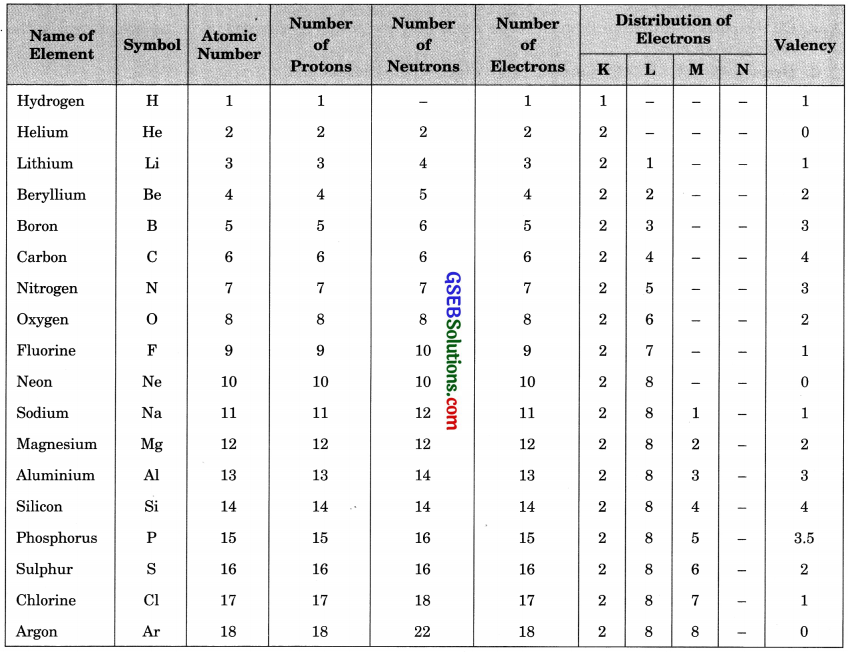# GSEB Solutions Class 9 Science Chapter 4 Structure of the Atom

Gujarat Board GSEB Textbook Solutions Class 9 Science Chapter 4 Structure of the Atom Textbook Questions and Answers, Notes Pdf.

## Gujarat Board Textbook Solutions Class 9 Science Chapter 4 Structure of the Atom

### Gujarat Board Class 9 Science Structure of the Atom InText Questions and Answers

Page – 47

Question 1.
What are canal rays?
Canal rays are positively charged radiations. These rays consist of positively charged particles known as protons. They were discovered by Goldstein in 1886.Question 2.
If an atom contains one electron and one proton, will it carry any charge or not?
An electron is a negatively charged particle, whereas a proton is a positively charged particle. The magnitude of their charges is equal. Therefore, an atom containing one electron and one proton will not carry any charge. Thus, it will be a neutral atom.Page – 49

Question 3.
On the basis of Thomson’s model of an atom, explain how the atom is neutral as a whole.
According to Thomson’s model of the atom, an atom consists of both negatively and positively charged particles. The negatively charged particles are embedded in the positively charged sphere. These negative and positive charges are equal in magnitude. Thus, by counterbalancing each other’s effect, they make an atom neutral.

Question 4.
On the basis of Rutherford’s model of an atom, which sub-atomic particle is pressent in the nucleus of an atom?
On the basis of Rutherford’s model of an atom, protons (positively charged particles) are present in the nucleus of an atom.

Question 5.
Draw a sketch of Bohr’s model of an atom with three shells.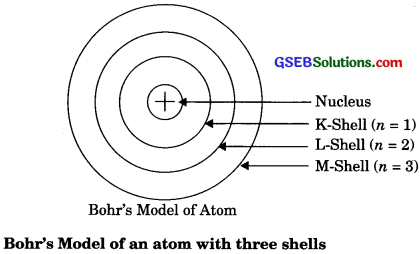Question 6.
What do you think would be the observation if the a-particle scattering experiment is carried out using a foil of a metal other than gold?
If the a-scattering experiment is carried out using a foil of a metal other than gold, there would be no change in the observation. In the a-scattering experiment, a gold foil was taken because gold is malleable and a thin foil of gold can be easily made. It is difficult to make such foils from other metals.

Page – 49

Question 7.
Name the three sub-atomfcc particles of an atom.
Electron, proton and neutron.

Question 8.
Helium atom has an atomic mass of 4u and two protons in its nucleus. How many neutrons does it have?
Number of neutrons = Atomic mass – Number of potons.
Therefore, the number of neutrons in the atom = 4 – 2 = 2.Page – 50

Question 9.
Write the distribution of electrons in carbon and sodium atoms.
(i) Atomic number of carbon = 6, i.e., total number of electrons = 6. Hence 1st-shell (K = shell) will have 2 electrons and the remaining electrons, i.e., 4 will be present in the 2nd shell, i.e., L-shell. Thus the distribution will be:
K L
2 4

(ii) Atomic number of sodium = 11, i.e., total number of electrons = 11. Hence, distribution will be
K L M
2 8 1.

Question 10.
If K and L shells of an atom are full, then what would be the total number of electrons in the atom?
The maximum capacity of K shell is 2 electrons and L shell can accommodate a maximum 8 electrons in it. Therefore, there will be ten electrons in the atom.

Page – 52

Question 11.
How will you find the valency of chlorine, sulphur and magnesium?
(i) Atomic number of chlorine = 17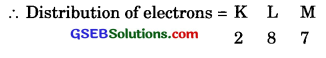Thus, outermost shell has 7 electrons. It can easily complete its octect by gaining one electron. Hence, its valency = 1.

(ii) Atomic number of sulphur = 16
∴ Distribution of electrons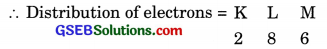Thus, outermost shell has 6 electrons.
Hence, valency = 8 – 6 = 2.

(iii) Atomic number of magnesium = 12.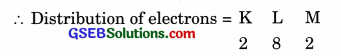Thus, outermost shell has only 2 electrons. It can easily lose these two electrons to complete its octect.
Hence, its valency = 2.

Page – 52

Question 12.
If number of electrons in an atom is 8 and number of protons is also 8, then
(i) What is the atomic number of the atom? and
(ii) What is the charge on the atom?
(i) The atomic number is equal to the number of protons. Therefore, the atomic number of the atom is 8.

(ii) Since the number of both electrons and protons is equal, therefore, the charge on the atom is 0.

Question 13.
With the help of table 4.1(Textbook Page 51), find out the mass of number of oxygen and sulphur atom.
Mass number of oxygen = Number of protons + Number of neutrons
= 8 + 8
= 16
Mass number of sulphur = Number of protons + Number of neutrons
= 16 + 16
= 32

Page – 53

Question 14.
For the symbol H, D and T tabulate three sub-atomic particles found in each of them.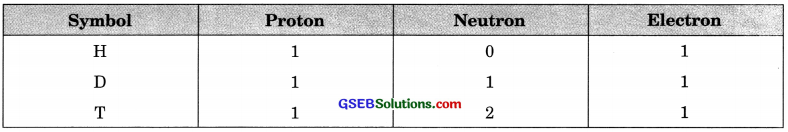Question 15.
Write the electronic configuration of any one pair of isotopes and isobars.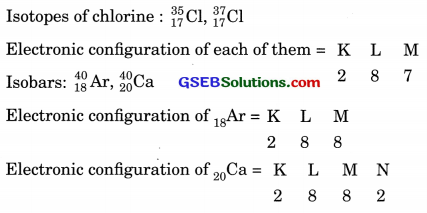### Gujarat Board Class 9 Science Structure of the Atom Textbook Questions and Answers

Question 1.
Compare the properties of electrons, protons and neutrons.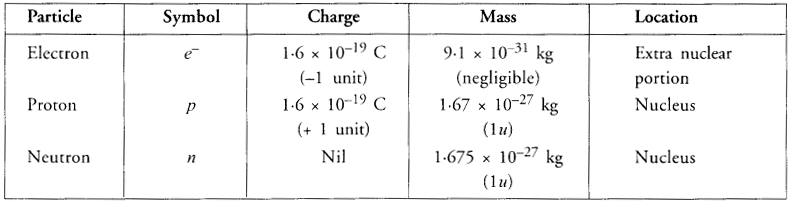Question 2.
What are the limitations of J.J. Thomson’s model of an atom ?
Limitations of Thomson’s model of an atom
(i) It could not explain the result of the scattering experiment performed by Rutherford.
(ii) It did not have any experimental evidence in its support.

Question 3.
What are the limitations of Rutherford’s model of an atom ?
Limitations of Rutherford Model atom

1. Rutherford model of atom could not explain the stability of the atom.
2. Rutherford model of atom could not explain as to how the electrons are distributed in the extra nuclear portion in an atom.

Question 4.
Describe Bohr’s model of an atom.
In 1913, Neils Bohr gave a theory regarding the distribution of electrons in the extra nuclear space in an atom. The main postulates of the theory are listed :

1. In the extra nuclear portion of an atom, the electrons revolve in well defined circular paths known as orbits.
2. These circular orbits are also known as energy levels or energy shells.
3. These have been designated as K, L, M, N, O, … (or as 1, 2, 3, 4, 5, …) based on the energy present.
4. The order of the energy of these energy shells is :
K<L<M<N<0 <…. or 1< 2< 3 < 4<5 <….
5. While revolving in an orbit, the electron is not in a position to either lose or gain energy. In other words, its energy remains stationary. Therefore, these energy states for the electrons are also known as stationary states.

Question 5.
Compare all the proposed models of an atom given in this chapter.
For Thomson’s Model of air atom:

1. It could not explain the result of the scattering experiment performed by Rutherford.
2. It did not have any experimental evidence in its support.

For Rutherford’s Model of an atom:

1. Rutherford model of atom could not explain the stability of the atom.
2. Rutherford model of atom could not explain as to how the electrons are distributed in the extra nuclear portion in an atom.

For Bohr’s Model of air atom:

1. It gave no idea about the shapes of the molecules formed by the combination of atoms.
2. According to Bohr’s theory, an electron in an atom follows a well defined circular path called orbit. However, the later studies have revealed that the path of the electron cannot be followed exactly. It is only of probable nature and not exact as stated by Bohr. However, it is not possible to discuss this concept at the present level of the students.

Question 6.
Summarise the rules for writing of distribution of electrons in various shells for the first eighteen elements.
The rules for writing of the distribution of electrons in various shells for the first eighteen elements are given below.
Following rules are followed to fill electrons in different energy levels.
(i) If n is the given number of orbit or energy level, then 2n2 gives the maximum number of electrons possible in a given orbit or energy level. Thus
First orbit or K-shell will have 2n2 = 2 x 22 = 2 electrons
Second orbit or L-shell will have 2n2 = 2 x 22 = 8 electrons
Third orbit or M-shell will have 2n2 = 2 x 32 = 18 electrons

(ii) If it is the outermost orbit, then it should not have more than 8 electrons.

(iii) There should be stepwise filling of electrons in different orbits, i.e., electrons are not accommodated in a given orbit if the earlier orbits or shells are incompletely filled.Question 7.
Define valency by taking examples of silicon and oxygen.
Valency is defined as the number of electrons which an atom can lose or gain or share with other atom/atoms so as to complete its octet, i.e., 8 electrons in the outermost shell.
Examples:
(i) Silicon: Atomic number of silicon = 14
∴ No. of electrons present = 14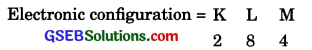Thus, outermost shell has 4 electrons which it can share with other atoms to complete its orbit.
Hence, its valency = 4.

(ii) Oxygen: Atomic number of oxygen = 8
∴ No. of electrons present = 8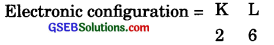Thus, outermost shell has 6 electrons. It will gain 2 electrons to complete its octet. Hence, valency = 2.

Question 8.
Explain with the examples
(i) Atomic number
(ii) Mass number
(iii) Isotopes and
(iv) Isobars.
Give any two uses of isotopes.
(i) Atomic number: The atomic number of an element is the total number of protons present in the atom of that element. For example, nitrogen has 7 protons in its atom. Thus, the atomic number of nitrogen is 7.

(ii)Mass number: The mass number of an element is the sum of the number of protons and neutrons present in the atom of that element. For example, the atom of boron has 5 protons and 6 neutrons. So, the mass number of boron is 5 + 6 = 11.

(iii) Isotopes: They are atoms of the same element and have same atomic number but different mass number/atomic mass. For example, Carbon: 126C and 146C.

(iv) Isobars: They are atoms of different elements having same mass number but different atomic numbers. For example, calcium, atomic number 20 and argon, atomic number 18 are isobars and have mass number 40.

The number of electrons in these atoms is different, but the mass number of both these elements is 40. That is, the total number of neutrons is the same in the atoms of this pair of element.

Two uses of isotopes are as follows:

• An isotope of uranium is used as a fuel in nuclear reactors.
• An isotope of cobalt is used in the treatment of cancer.

Question 9.
Na+ has completely filled K and L shells. Explain.
The atomic number of sodium is 11. So neutral sodium atom has 11 electrons. Na+ has 10 electrons. Out of 10, K-shell contains 2 electrons and L-shell 8 electrons. Thus, Na+ has completely filled K and L-shells.

Question 10.
If bromine atom is available in the form of, say, two isotopes $$_{ 35 }^{ 79 }{ Br }$$ and $$_{35}^{81}$$(50.3%). Calculate the average atomic mass of bromine atom.
% of Br isotope with mass number 79 = 49.7
% of Br isotope with mass number 81 = 50.3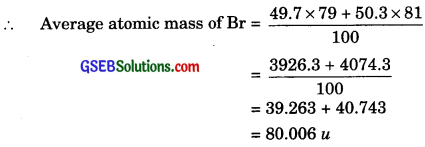Question 11.
The average atomic mass of a sample of an element X is 16.2 u. What are the percentages of isotopes $$_{ 16 }^{ 8 }{ X }$$ and $$_{ 18 }^{ 8 }{ X }$$ in the sample?
Let the percentage of $$_{ 16 }^{ 8 }{ X }$$ be A.
Then the percentage of $$_{ 18 }^{ 8 }{ X }$$ = 100 – A
Then, we have
$$\frac { 16 × A }{ 100 }$$ + $$\frac { (100 – A) × 18}{ 2 }$$
or A = 90
$$_{ 16 }^{ 8 }{ X }$$ is 90% and $$_{ 18 }^{ 8 }{ X }$$ is 10%.

Question 12.
If Z = 3, what would be the valency of the element? Also, name the element.
If Z = 3, i.e., atomic number is 3. Then element is lithium. It has distribution of electrons as 2, 1. Thus lithium has a valency of 1.

Question 13.
Composition of the nuclei of two atomic species X and Y are given as under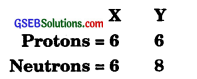Give the mass numbers of X and Y. What is the relation between the two species?
Mass number of X = Number of protons + Number of neutrons = 6 + 6 = 12
Mass number of Y = Number of protons + Number of neutrons = 6 + 8 = 14
These two atomic species X and Y have the same atomic number, but different mass numbers. Hence, they are isotopes.Question 14.
For the following statements, write T for True and F for False.
(a) J. J. Thomson proposed that the nucleus of an atom contains only nucleons.
(b) A neutron is formed by an electron and a proton combining together.
(c) The mass of an electron is about 1/2000 times that of a proton.
(d) Isotope of iodine is used for making tincture iodine, which is used as a medicine.
(a) False
(b) False
(c) True
(d) False

Question 15.
Rutherford’s alpha-particle scattering experiment was responsible for the discovery of
(a) Atomic nucleus
(b) Electron
(c) Proton
(d) Neutron
(a) Atomic nucleus

Question 16.
Isotopes of an element have
(a) the same physical properties
(b) different chemical properties
(c) different number of neutrons
(d) different atomic numbers
(c) different number of neutrons

Question 17.
Number of valence electrons in Cl ion is:
(a) 16
(b) 8
(c) 17
(d) 18
(b) 8

Question 18.
Which one of the following is a correct electronic configuration of sodium?
(a) 2, 8
(b) 8, 2, 1
(c) 2, 1, 8
(d) 2, 8, 1
(d) 2,8, 1

Question 19.
Complete the following table.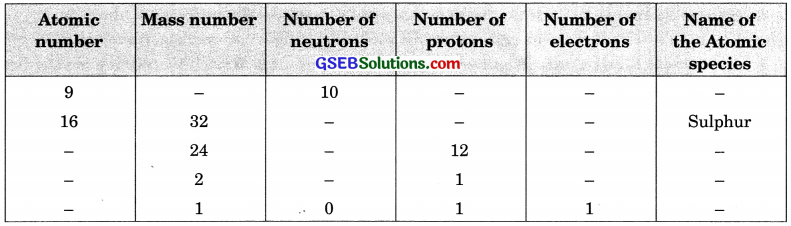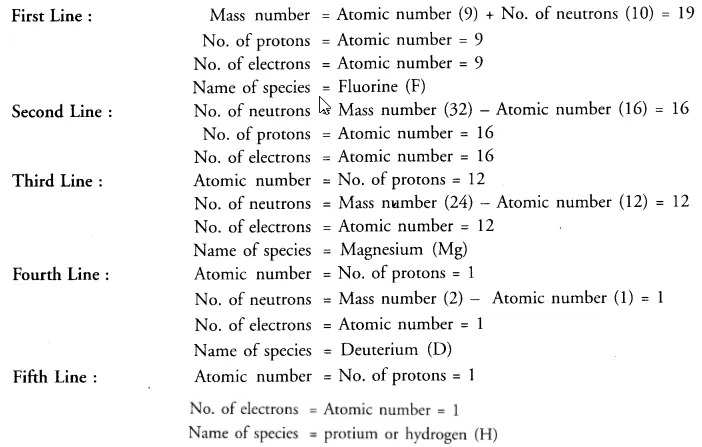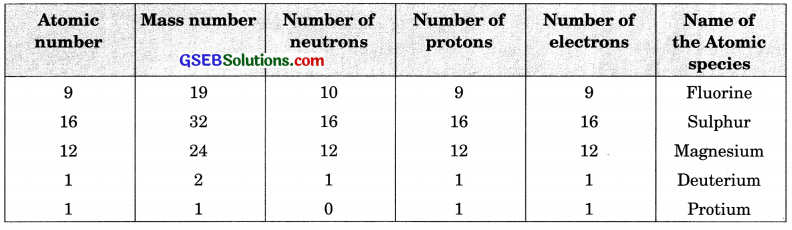In-Text Activities Solved

(Textbook Page 46)

Activity 4.1